# Hardest colleges to get into in Nebraska

Top 7 colleges in Nebraska with the lowest acceptance rates
What are the hardest colleges to get into in Nebraska? We've got you covered. We've compiled a national college database and have created a list of the hardest universities to get into in Nebraska below. These are the hardest 4 year colleges to get into in Nebraska, and sometimes some of the hardest colleges to get into in the US. You could even say these are the best colleges in Nebraska. We also include each college's average SAT and ACT scores so that you can see where you're most competitive. Read on to find out more.

## Bryan College of Health Sciences acceptance rate

Bryan College of Health Sciences acceptance rate is 40%.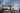The average SAT score for Bryan College of Health Sciences is 1110.

• SAT composite: 1110
• SAT math: 555

The average ACT score for Bryan College of Health Sciences is 22.

## Union College acceptance rate

Union College acceptance rate is 59.9%.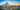The average SAT score for Union College is 1063.

• SAT composite: 1063
• SAT math: 510

The average ACT score for Union College is 23.

## University of Nebraska Omaha acceptance rate

University of Nebraska Omaha acceptance rate is 70.8%.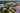The average SAT score for University of Nebraska Omaha is 1140.

• SAT composite: 1140
• SAT math: 570

The average ACT score for University of Nebraska Omaha is 23.

## Creighton University acceptance rate

Creighton University acceptance rate is 71.4%.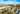The average SAT score for Creighton University is 1254.

• SAT composite: 1254
• SAT math: 630

The average ACT score for Creighton University is 27.

## Concordia University Nebraska acceptance rate

Concordia University Nebraska acceptance rate is 75%.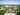The average SAT score for Concordia University Nebraska is 1017.

• SAT composite: 1017
• SAT math: 500

The average ACT score for Concordia University Nebraska is 23.

## University of Nebraska at Lincoln acceptance rate

University of Nebraska at Lincoln acceptance rate is 79.6%.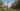The average SAT score for University of Nebraska at Lincoln is 1240.

• SAT composite: 1240
• SAT math: 620

The average ACT score for University of Nebraska at Lincoln is 25.

## University of Nebraska at Kearney acceptance rate

University of Nebraska at Kearney acceptance rate is 81.7%.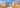The average SAT score for University of Nebraska at Kearney is 1090.

• SAT composite: 1090
• SAT math: 560